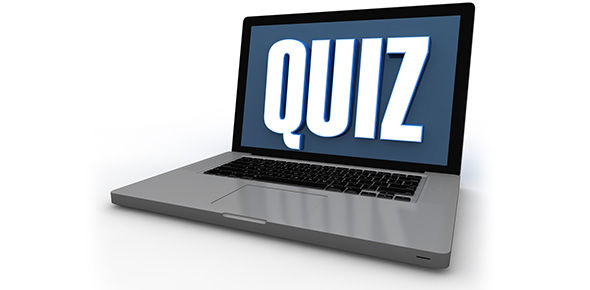# I.E.D Warm-up Quiz #5

10 Questions | Total Attempts: 39SettingsSept. 24 - Sept. 28

• 1.
Find the odds of rolling a number less than or equal to three on a number cube.
• A.

1/2

• B.

1/1

• C.

1/3

• D.

5/6

• 2.
If you have a standard 52-card deck of playing cards, what is the probability of drawing the king of hearts?
• A.

1/4

• B.

1/13

• C.

1/26

• D.

1/52

• 3.
What is the domain of the following set: {(8,7), (4,3), (-3,7), (0, -6)}
• A.

{-3,0,4,8}

• B.

{-6,3,7}

• C.

{1,1,10,-6}

• D.

{-6,-3,0,3,4,7,7,8}

• 4.
Simplify:      4y + 7x + 3y - 4x
• A.

3x + 7y

• B.

-28x + 12y

• C.

3x7y

• D.

21xy

• 5.
If you have a standard 52-card deck of playing cards, what is the probability of drawing a jack?
• A.

1/4

• B.

1/13

• C.

1/26

• D.

1/52

• 6.
Solve:     23 - 2x  = 49
• A.

X = 13

• B.

X = -13

• C.

X = 36

• D.

X = -36

• 7.
A bag contains 18 blue marbles, 23 purple marbles, 10 white marbles, and 9 red marbles. What is the probability of randomly selecting a marble that is not blue?
• A.

7/10

• B.

3/10

• C.

1/4

• D.

1/10

• 8.
If you have a standard 52-card deck of playing cards, what is the probability of drawing a seven or a heart?
• A.

3/52

• B.

17/52

• C.

3/13

• D.

4/13

• 9.
The equation of a line that contains the points ( 8, -3) and ( -2, -7) has a slope of m = ___.
• A.

2/5

• B.

-9/11

• C.

5/2

• D.

-2/5

• 10.
Which ordered pair is the solution to the given system of equations? x - 3y = 9 x - 2y = 5
• A.

(-3, -4)

• B.

(9, -3)

• C.

(-6, 1)

• D.

(3, -2)Back to top